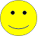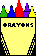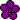# Math Practice Topic: Commutative Property 2

 Description: Completion of equations that demonstrate the commutative property using multiplication. For example, since 3*5=15, 5*3=15. Adaptive Learning Progression: Starts with visuals, then builds up a deep understanding using just equations. Start using MathScore for free

## Sample Levels (out of 4)

Write the equation for the commutative property of multiplication shown by the picture.

1.=Equation:
2.=Equation:
3.=Equation:

 1 If 4 × 9 = 36, then 9 × 4 = 2 Rewrite the expression 9 × 3 using the commutative property. 3 4 × 1 = × 4 If 7 × 3 = 21, then 3 × 7 =

1.
Which equation(s) show the commutative property?
 10 ÷ 3 = 3 ÷ 10
 3 + 5 + 9 = 5 + 3
 4 + 7 = 4 + 7
 1 + 4 = 5 + 0
 none of the above
2.
Rewrite the expression 21 × 25 using the commutative property.
3.
Which equation(s) show the commutative property?